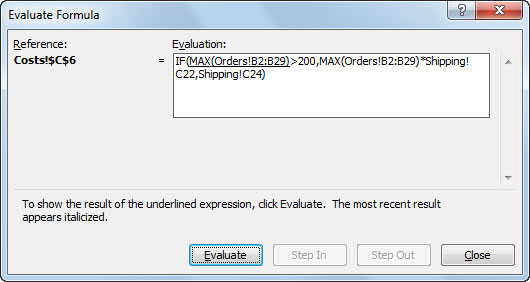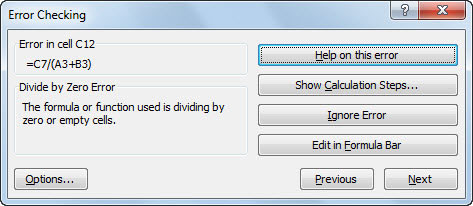##### Excel 2010 Just the Steps For DummiesThe Evaluate Formula dialog box in Excel 2010 walks you through the steps used in calculating a result from a formula. This helps locate errors in formulas that are long or have precedents. For example, the formula below refers to different worksheets. Using the Evaluate Formula dialog box makes it easy to see how this formula is worked out by Excel. The step-by-step approach lets you see what is done at each step.

`=IF(MAX(Orders!B2:B29)>200,MAX(Orders!B2:B29)*Shipping!C22,Shipping!C24)`

## Evaluating formulas

Follow these steps to evaluate a formula using the Evaluate Formula dialog box:

1. Select the cell containing the formula and click the Evaluate Formula button on the Formulas tab of the Ribbon.

The Evaluate Formula dialog box appears.Using the Evaluate Formula dialog box to assess a formula.
2. Click the Evaluate button one or more times to evaluate each expression in the formula.

With each successive click of the Evaluate button, the Evaluate Formula dialog box displays the interim results. The Step In and Step Out buttons are enabled during the steps that work on the precedents.

The Evaluate Formula dialog box is great for really seeing how each little step feeds into the final calculated result. Using this dialog box lets you pinpoint exactly where a complex formula has gone sour.

## Checking errors

A similar error-hunting tool is the Error Checking dialog box. To use this tool, follow these steps:

1. Select the cell containing the formula and click the Error Checking button on the Formulas tab.

The Error Checking dialog box appears.Checking the cause of an error.
2. Click any of the following buttons to help analyze the error and make decisions about how to correct it:

• Help On This Error displays the Excel Help window.

• Show Calculation Steps opens the Evaluate Formula dialog box.

• Ignore Error ensures that Excel no longer cares about the error. The cell may still display an error symbol, but Excel does not give a hoot, and probably you won’t either, since you clicked the button.

• Edit in Formula Bar places the cursor in the Formula Bar, making it easy for you to edit the formula.

• Options opens the Excel Options dialog box.

• Previous and Next cycle through the multiple errors on the worksheet, assuming there is more than one error.

The Error Checking drop-down list hosts the Trace Error command. Only precedents are pointed out by the tracer lines. This makes it easy to see the cells that feed into a cell that has an error.